4. STELLAR BARYON BUDGET

With the help of some simple stellar population synthesis tools it is possible at this stage to make an estimate of the stellar mass density that produced the integrated light observed today. The total bolometric luminosity of a simple stellar population (a single generation of coeval stars) having mass M can be well approximated by a power-law with time for all ages t100 Myr,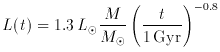(1)

(cf. ), where we have assumed solar metallicity and a Salpeter IMF truncated at 0.1 and 125 M. In a stellar system with arbitrary star-formation rate per unit cosmological volume,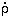*, the comoving bolometric emissivity at time t is given by the convolution integral(2)

The total background light observed at Earth (t = tH) is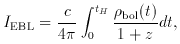(3)

where the factor (1 + z) at the denominator is lost to cosmic expansion when converting from observed to radiated (comoving) luminosity density. From the above equations it is easy to derive in a EdS cosmology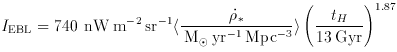(4)

The observations shown in Figure 1 therefore imply a ``fiducial'' mean star formation density of <* > = 0.054 I40 Myr-1 Mpc-3. In the instantaneous recycling approximation, the total stellar mass density observed today is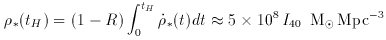(5)

(corresponding to* = 0.007 I40), where R is the mass fraction of a generation of stars that is returned to the interstellar medium, R0.3 for a Salpeter IMF. The optical/FIR background therefore requires that about 10% of the nucleosynthetic baryons today  are in the forms of stars and their remnants. The predicted stellar mass-to-blue light ratio is < M / LB >5. These values are quite sensitive to the lower-mass cutoff of the IMF, as very-low mass stars can contribute significantly to the mass but not to the integrated light of the whole stellar population. A lower cutoff of 0.5 Minstead of the 0.1 Madopted would decrease the mass-to-light ratio (and*) by a factor of 1.9 for a Salpeter function.